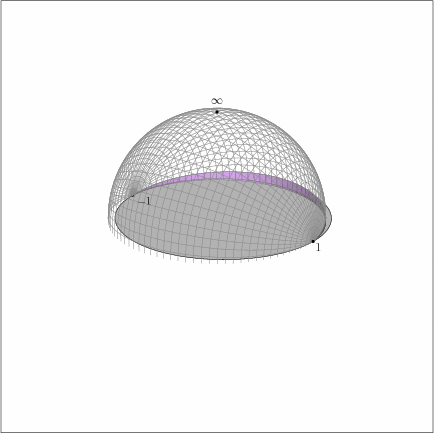A Riemann surface is a “universe” locally modelled on open sets…A

Riemann surface

is a “universe” locally modelled on open sets in the complex plane, and equipped with extra structure so that complex analysis can be done. Simple examples include

Polynomial equations in two variables define special Riemann surfaces, called plane curves, such as

In the seminar, we’ll review tools from complex analysis, including differential forms, contour integration, the Cauchy integral formula, and residues. We’ll learn how to “build” Riemann surfaces by (i) “gluing” together pieces of the complex plane, (ii) analytic continuation, and (iii) “dividing” an existing Riemann surface by the action of a group. We’ll study the local and global behavior of holomorphic maps between Riemann surfaces. Topological covering maps and mappings between plane curves defined by projection will furnish particularly interesting examples.

If time and student interest permit, we’ll study the interrelationship between complex analytic structure, topology, and differential geometry embodied by the Gauss-Bonnet and Uniformization theorems. All necessary background material from differential geometry will be covered in the seminar.